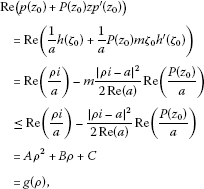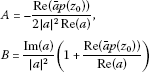# On sufficient conditions for Carathéodory functions with applications

## Abstract

In the present paper, we derive some interesting relations associated with the Carathéodory functions which yield sufficient conditions for the Carathéodory functions in the open unit disk $\mathbb{U}=\left\{z:|z|<1\right\}$. Some interesting applications of the main results are also obtained.

MSC:30C45, 30C80.

## 1 Introduction

Let P denote the class of functions of the form

$p\left(z\right)=\sum _{n=0}^{\mathrm{\infty }}{p}_{n}{z}^{n},$

which are analytic in the unit disc $\mathbb{U}=\left\{z:|z|<1\right\}$. The function $p\left(z\right)$ is called a Carathéodory function if it satisfies the condition

$Re\left(p\left(z\right)\right)>0.$

Moreover, let A denote the class of functions of the form

$f\left(z\right)=z+\sum _{n=2}^{\mathrm{\infty }}{a}_{n}{z}^{n},$
(1.1)

which are analytic in the unit disc $\mathbb{U}$.

A function $f\left(z\right)\in A$ is in K, the class of convex functions, if it satisfies

$Re\left(1+\frac{z{f}^{\mathrm{\prime }\mathrm{\prime }}\left(z\right)}{f\left(z\right)}\right)>0\phantom{\rule{1em}{0ex}}\left(z\in \mathbb{U}\right).$
(1.2)

Also, a function $f\left(z\right)\in A$ is in ${S}^{\lambda }$ ($|\lambda |<\frac{\pi }{2}$), the class of λ-spirallike functions, if it satisfies

$Re\left({e}^{i\lambda }\frac{z{f}^{\mathrm{\prime }}\left(z\right)}{f\left(z\right)}\right)>0\phantom{\rule{1em}{0ex}}\left(z\in \mathbb{U}\right).$
(1.3)

Moreover, we denote by ${S}^{\ast }={S}^{0}$ the class of starlike functions in $\mathbb{U}$.

Definition 1.1 Let $f\left(z\right)$ and $F\left(z\right)$ be analytic functions. The function $f\left(z\right)$ is said to be subordinate to $F\left(z\right)$, written $f\left(z\right)\prec F\left(z\right)$, if there exists a function $w\left(z\right)$ analytic in $\mathbb{U}$, with $w\left(0\right)=0$ and $|w\left(z\right)|\le 1$, and such that $f\left(z\right)=F\left(w\left(z\right)\right)$. If $F\left(z\right)$ is univalent, then $f\left(z\right)\prec F\left(z\right)$ if and only if $f\left(0\right)=F\left(0\right)$ and $f\left(\mathbb{U}\right)\subset F\left(\mathbb{U}\right)$.

Definition 1.2 Let $\mathbb{D}$ be the set of analytic functions $q\left(z\right)$ and injective on $\overline{\mathbb{U}}\mathrm{\setminus }E\left(q\right)$, where

$E\left(q\right)=\left\{\zeta \in \partial \mathbb{U}:\underset{z\to \zeta }{lim}q\left(z\right)=\mathrm{\infty }\right\}$

and ${q}^{\mathrm{\prime }}\left(\zeta \right)\ne 0$ for $\zeta \in \partial \mathbb{U}\mathrm{\setminus }E\left(q\right)$. Further, let ${\mathbb{D}}_{a}=\left\{q\left(z\right)\in \mathbb{D}:q\left(0\right)=a\right\}$.

Many authors have obtained several relations of Carathéodory functions, e.g., see ().

In the present paper, we derive some relations associated with the Carathéodory functions which yield the sufficient conditions for Carathéodory functions in $\mathbb{U}$. Some applications of the main results are also obtained.

## 2 Main results

To prove our results, we need the following lemma due to Miller and Mocanu [, p.24]

Lemma 2.1 Let $q\left(z\right)\in {\mathbb{D}}_{a}$ and let

$p\left(z\right)=b+{b}_{n}{z}^{n}+\cdots$
(2.1)

be analytic in $\mathbb{U}$ with $p\left(z\right)\ne b$. If $p\left(z\right)\nprec q\left(z\right)$, then there exist points ${z}_{0}\in \mathbb{U}$ and ${\zeta }_{0}\in \partial \mathbb{U}\mathrm{\setminus }E\left(q\right)$ and on $m\ge n\ge 1$ for which

1. (i)

$p\left({z}_{0}\right)=q\left({\zeta }_{0}\right)$,

2. (ii)

${z}_{0}{p}^{\mathrm{\prime }}\left({z}_{0}\right)=m{\zeta }_{0}{q}^{\mathrm{\prime }}\left({\zeta }_{0}\right)$.

Theorem 2.1 Let

$P:\mathbb{U}\to \mathbb{C}$

with

$Re\left(\overline{a}P\left(z\right)\right)>0\phantom{\rule{1em}{0ex}}\left(a\in \mathbb{C}\right).$

If $p\left(z\right)$ is an analytic function in $\mathbb{U}$ with $p\left(0\right)=1$ and

$Re\left(p\left(z\right)+P\left(z\right)z{p}^{\mathrm{\prime }}\left(z\right)\right)>\frac{E}{2|a{|}^{2}Re\left(\overline{a}P\left(z\right)\right)},$
(2.2)

then

$Re\left(ap\left(z\right)\right)>0,$

where

$E=-\left(Re\left(a\right)\right){\left(Re\left(\overline{a}P\left(z\right)\right)\right)}^{2}+2Re\left(\overline{a}P\left(z\right)\right){\left(Im\left(a\right)\right)}^{2}+\left(Re\left(a\right)\right){\left(Im\left(a\right)\right)}^{2}$
(2.3)

with $Re\left(a\right)>0$.

Proof Let us define both $q\left(z\right)$ and $h\left(z\right)$ as follows:

$q\left(z\right)=ap\left(z\right)$

and

$h\left(z\right)=\frac{a+\overline{a}z}{1-z}\phantom{\rule{1em}{0ex}}\left(Re\left(a\right)>0\right),$

where $p\left(z\right)$ is defined by (2.1) since $q\left(z\right)$ and $h\left(z\right)$ are analytic functions in $\mathbb{U}$ with $q\left(0\right)=h\left(0\right)=a\in \mathbb{C}$ with

$h\left(\mathbb{U}\right)=\left\{w:Re\left(w\right)>0\right\}.$

Now, we suppose that $q\left(z\right)\nprec h\left(z\right)$. Therefore, by using Lemma 2.1, there exist points

${z}_{0}\in \mathbb{U}\phantom{\rule{1em}{0ex}}\text{and}\phantom{\rule{1em}{0ex}}{\zeta }_{0}\in \partial \mathbb{U}\mathrm{\setminus }\left\{1\right\}$

such that $q\left({z}_{0}\right)=h\left({\zeta }_{0}\right)$ and ${z}_{0}{q}^{\mathrm{\prime }}\left({z}_{0}\right)=m{\zeta }_{0}{h}^{\mathrm{\prime }}\left({\zeta }_{0}\right)$, $m\ge n\ge 1$.

We note that

${\zeta }_{0}={h}^{-1}\left(q\left({z}_{0}\right)\right)=\frac{q\left({z}_{0}\right)-a}{q\left({z}_{0}\right)+\overline{a}}$
(2.4)

and

${\varsigma }_{0}{h}^{\mathrm{\prime }}\left({\varsigma }_{0}\right)=-\frac{|q\left({z}_{0}\right)-a{|}^{2}}{2Re\left(a-q\left({z}_{0}\right)\right)}.$
(2.5)

We have $h\left({\zeta }_{0}\right)=\rho i$ ($\rho \in \mathbb{R}$); therefore,(2.6)

whereand

$C=-\frac{Re\left(\overline{a}p\left({z}_{0}\right)\right)}{2Re\left(a\right)}.$

We can see that the function $g\left(\rho \right)$ in (2.6) takes the maximum value at ${\rho }_{1}$ given by

${\rho }_{1}=Im\left(a\right)\left(1+\frac{Re\left(a\right)}{Re\left(\overline{a}p\left({z}_{0}\right)\right)}\right).$

Hence, we have

$\begin{array}{rcl}Re\left(p\left({z}_{0}\right)+P\left({z}_{0}\right)z{p}^{\mathrm{\prime }}\left({z}_{0}\right)\right)& \le & g\left({\rho }_{1}\right)\\ =& \frac{E}{2|a{|}^{2}Re\left(\overline{a}P\left(z\right)\right)},\end{array}$

where E is defined by (2.3). This is a contradiction to (2.2). Then we obtain $Re\left(ap\left(z\right)\right)>0$. □

Theorem 2.2 Let $p\left(z\right)$ be a nonzero analytic function in $\mathbb{U}$ and $p\left(0\right)=1$. If

${\gamma }_{1}
(2.7)

where

${\gamma }_{1}=-\frac{\sqrt{|a{|}^{2}+2{\left(Re\left(a\right)\right)}^{2}}-Im\left(a\right)}{Rea}$

and

${\gamma }_{2}=\frac{\sqrt{|a{|}^{2}+2{\left(Re\left(a\right)\right)}^{2}}+Im\left(a\right)}{Re\left(a\right)},$

then

$Re\left(ap\left(z\right)\right)>0,$

where $Re\left(a\right)>0$.

Proof Let us define both $q\left(z\right)$ and $h\left(z\right)$ as follows:

$q\left(z\right)=ap\left(z\right)$

and

$h\left(z\right)=\frac{a+\overline{a}z}{1-z}\phantom{\rule{1em}{0ex}}\left(Re\left(a\right)>0\right),$

where $p\left(z\right)$ is defined by (2.1) since $q\left(z\right)$ and $h\left(z\right)$ are analytic functions in $\mathbb{U}$ with $q\left(0\right)=h\left(0\right)=a\in \mathbb{C}$ with

$h\left(\mathbb{U}\right)=\left\{w:Re\left(w\right)>0\right\}.$

Now, we suppose that $q\left(z\right)\nprec h\left(z\right)$. Therefore, by using Lemma 2.1, there exist points

${z}_{0}\in \mathbb{U}\phantom{\rule{1em}{0ex}}\text{and}\phantom{\rule{1em}{0ex}}{\zeta }_{0}\in \partial \mathbb{U}\mathrm{\setminus }\left\{1\right\}$

such that $q\left({z}_{0}\right)=h\left({\zeta }_{0}\right)$ and ${z}_{0}{q}^{\mathrm{\prime }}\left({z}_{0}\right)=m{\zeta }_{0}{h}^{\mathrm{\prime }}\left({\zeta }_{0}\right)$, $m\ge n\ge 1$.

We note that

${\zeta }_{0}{h}^{\mathrm{\prime }}\left({\zeta }_{0}\right)=-\frac{|q\left({z}_{0}\right)-a{|}^{2}}{2Re\left(a-q\left({z}_{0}\right)\right)}.$
(2.8)

We have $h\left({\zeta }_{0}\right)=\rho i$ ($\rho \in \mathbb{R}$); therefore,

$\begin{array}{rcl}Im\left(p\left({z}_{0}\right)+\frac{{z}_{0}{p}^{\mathrm{\prime }}\left({z}_{0}\right)}{p\left({z}_{0}\right)}\right)& =& Im\left(q\left({z}_{0}\right)+\frac{{z}_{0}{q}^{\mathrm{\prime }}\left({z}_{0}\right)}{q\left({z}_{0}\right)}\right)\\ =& Im\left(\frac{h\left({\zeta }_{0}\right)}{a}+\frac{m{\zeta }_{0}{h}^{\mathrm{\prime }}\left({\zeta }_{0}\right)}{h\left({\zeta }_{0}\right)}\right)\\ =& Im\left(\frac{\rho i}{a}-\frac{m|\rho i-a{|}^{2}}{2Re\left(a\right)\rho i}\right)\\ =& \frac{\rho }{|a{|}^{2}}Re\left(a\right)+\frac{m|\rho i-a{|}^{2}}{2\rho Re\left(a\right)}.\end{array}$

For the case $\rho >0$, we obtain

$\begin{array}{rcl}Im\left(p\left({z}_{0}\right)+\frac{{z}_{0}{p}^{\mathrm{\prime }}\left({z}_{0}\right)}{p\left({z}_{0}\right)}\right)& \ge & \frac{\rho }{|a{|}^{2}}Re\left(a\right)+\frac{|\rho i-a{|}^{2}}{2\rho Re\left(a\right)}\\ =& \frac{1}{2\rho Re\left(a\right)}\left[\left(1+2{\left(\frac{Re\left(a\right)}{|a|}\right)}^{2}\right){\rho }^{2}+2Im\left(a\right)\rho +|a|\right]\\ =& g\left(\rho \right).\end{array}$
(2.9)

We can see that the function $g\left(\rho \right)$ in (2.9) takes the minimum value at ${\rho }_{1}$ given by

${\rho }_{1}=\frac{|a{|}^{2}}{\sqrt{|a{|}^{2}+2{\left(Re\left(a\right)\right)}^{2}}}.$

Hence, we have

$\begin{array}{rcl}Im\left(p\left({z}_{0}\right)+\frac{{z}_{0}{p}^{\mathrm{\prime }}\left({z}_{0}\right)}{p\left({z}_{0}\right)}\right)& \ge & g\left({\rho }_{1}\right)\\ =& {\gamma }_{2}.\end{array}$

This is a contradiction to (2.7). Then we obtain $Re\left(ap\left(z\right)\right)>0$.

For the case $\rho <0$, we obtain

$\begin{array}{rcl}Im\left(p\left({z}_{0}\right)+\frac{{z}_{0}{p}^{\mathrm{\prime }}\left({z}_{0}\right)}{p\left({z}_{0}\right)}\right)& \le & \frac{\rho }{|a{|}^{2}}Re\left(a\right)+\frac{|\rho i-a{|}^{2}}{2\rho Re\left(a\right)}\\ =& \frac{1}{2\rho Re\left(a\right)}\left[\left(1+2{\left(\frac{Re\left(a\right)}{|a|}\right)}^{2}\right){\rho }^{2}+2Im\left(a\right)\rho +|a{|}^{2}\right]\\ =& g\left(\rho \right).\end{array}$
(2.10)

We can see that the function $g\left(\rho \right)$ in (2.10) takes the maximum value at ${\rho }_{2}$ given by

${\rho }_{2}=-\frac{|a{|}^{2}}{\sqrt{|a{|}^{2}+2{\left(Re\left(a\right)\right)}^{2}}}.$

Hence, we have

$\begin{array}{rcl}Im\left(p\left({z}_{0}\right)+\frac{{z}_{0}{p}^{\mathrm{\prime }}\left({z}_{0}\right)}{p\left({z}_{0}\right)}\right)& \le & g\left({\rho }_{2}\right)\\ =& {\gamma }_{1}.\end{array}$

This is a contradiction to (2.7). Then we obtain $Re\left(ap\left(z\right)\right)>0$. □

Theorem 2.3 Let $p\left(z\right)$ be a nonzero analytic function in $\mathbb{U}$ with $p\left(0\right)=1$. If

$|p\left(z\right)+\frac{z{p}^{\mathrm{\prime }}\left(z\right)}{p\left(z\right)}-1|<\frac{3Re\left(a\right)}{2|a|},$

then

$Re\left(\frac{a}{p\left(z\right)}\right)>0,$

where $Re\left(a\right)>0$.

Proof Let us define both $q\left(z\right)$ and $h\left(z\right)$ as follows:

$q\left(z\right)=ap\left(z\right)$

and

$h\left(z\right)=\frac{a+\overline{a}z}{1-z}\phantom{\rule{1em}{0ex}}\left(Re\left(a\right)>0\right),$

where $p\left(z\right)$ is defined by (2.1) since $q\left(z\right)$ and $h\left(z\right)$ are analytic functions in $\mathbb{U}$ with $q\left(0\right)=h\left(0\right)=a\in \mathbb{C}$ with

$h\left(\mathbb{U}\right)=\left\{w:Rew>0\right\}.$

Now, we suppose that $q\left(z\right)\nprec h\left(z\right)$. Therefore, by using Lemma 2.1, there exist points

${z}_{0}\in \mathbb{U}\phantom{\rule{1em}{0ex}}\text{and}\phantom{\rule{1em}{0ex}}{\zeta }_{0}\in \partial \mathbb{U}\mathrm{\setminus }\left\{1\right\}$

such that $q\left({z}_{0}\right)=h\left({\zeta }_{0}\right)$ and ${z}_{0}{q}^{\mathrm{\prime }}\left({z}_{0}\right)=m{\zeta }_{0}{h}^{\mathrm{\prime }}\left({\zeta }_{0}\right)$, $m\ge n\ge 1$.

We note that

${\zeta }_{0}{h}^{\mathrm{\prime }}\left({\zeta }_{0}\right)=-\frac{|q\left({z}_{0}\right)-a{|}^{2}}{2Re\left(a-q\left({z}_{0}\right)\right)}.$
(2.11)

We have $h\left({\zeta }_{0}\right)=\rho i$ ($\rho \in \mathbb{R}$).

Therefore,

$\begin{array}{rcl}\frac{|p\left({z}_{0}\right)+\frac{z{p}^{\mathrm{\prime }}\left({z}_{0}\right)}{p\left({z}_{0}\right)}-1|}{|p\left({z}_{0}\right)|}& =& |\frac{\rho i}{a}-\frac{m}{a}\frac{|a-i\rho {|}^{2}}{2Re\left(a\right)}-1|\\ \ge & \frac{1}{|a|}|\frac{m|a-i\rho {|}^{2}}{2Re\left(a\right)}+Re\left(a\right)|\\ \ge & \frac{1}{|a|}\left(\frac{|a-i\rho {|}^{2}}{2Re\left(a\right)}+Re\left(a\right)\right)\\ \ge & \frac{1}{2|a|Re\left(a\right)}\left(3{\left(Re\left(a\right)\right)}^{2}+{\left(Im\left(a\right)-\rho \right)}^{2}\right)\\ \ge & \frac{3Re\left(a\right)}{2|a|}.\end{array}$

This is a contradiction to (2.7). Then we obtain $Re\left(\frac{a}{p\left(z\right)}\right)>0$. □

## 3 Applications and examples

Putting $P\left(z\right)=\beta$ ($\beta >0$; real) in Theorem 2.1, we have the following corollary.

Corollary 3.1 If $p\left(z\right)$ is an analytic function in $\mathbb{U}$ with $p\left(0\right)=1$ and

$Re\left(p\left(z\right)+\beta z{p}^{\mathrm{\prime }}\left(z\right)\right)>\frac{E}{2\beta |a{|}^{2}Re\left(a\right)},$

then

$Re\left(ap\left(z\right)\right)>0,$

where

$E=-\left(Re\left(a\right)\right)\left[{\beta }^{2}{\left(Re\left(a\right)\right)}^{2}+\left(1+2\beta \right){\left(Im\left(a\right)\right)}^{2}\right]$

with $Re\left(a\right)>0$.

Putting $\beta =1$ in Corollary 3.1, we obtain the following corollary.

Corollary 3.2 If $p\left(z\right)$ is an analytic function in $\mathbb{U}$ with $p\left(0\right)=1$ and

$Re\left(p\left(z\right)+z{p}^{\mathrm{\prime }}\left(z\right)\right)>\frac{3}{2}-2{\left(\frac{Re\left(a\right)}{|a|}\right)}^{2},$

then

$Re\left(ap\left(z\right)\right)>0,$

where $Re\left(a\right)>0$.

Putting $p\left(z\right)=\frac{f\left(z\right)}{g\left(z\right)}$ and $P\left(z\right)=\frac{g\left(z\right)}{z{g}^{\mathrm{\prime }}\left(z\right)}$ in Theorem 2.1, we have the following corollary.

Corollary 3.3 Let $f\left(z\right)\in A$, $g\left(z\right)\in {S}^{\ast }$ and

$Re\left(\frac{{f}^{\mathrm{\prime }}\left(z\right)}{{g}^{\mathrm{\prime }}\left(z\right)}\right)>\frac{3}{2}-2{\left(\frac{Re\left(a\right)}{|a|}\right)}^{2}.$

Then

$Re\left(a\frac{f\left(z\right)}{g\left(z\right)}\right)>0,$

where $Re\left(a\right)>0$.

Example 3.1 Let $f\left(z\right)\in A$ satisfy the following relation:

$Re\left({f}^{\mathrm{\prime }}\left(z\right)\right)>\frac{3}{2}-2{\left(\frac{Re\left(a\right)}{|a|}\right)}^{2}.$

Then

$Re\left(a\frac{f\left(z\right)}{z}\right)>0,$

where $Re\left(a\right)>0$.

Example 3.2 Let $f\left(z\right)\in A$ satisfy the following relation:

$Re\left(\left(2+\frac{z{f}^{\mathrm{\prime }\mathrm{\prime }}\left(z\right)}{{f}^{\mathrm{\prime }}\left(z\right)}-\frac{z{f}^{\mathrm{\prime }}\left(z\right)}{f\left(z\right)}\right)\frac{z{f}^{\mathrm{\prime }}\left(z\right)}{f\left(z\right)}\right)>\frac{3}{2}-2{\left(\frac{Re\left(a\right)}{|a|}\right)}^{2}.$

Then

$Re\left(a\frac{z{f}^{\mathrm{\prime }}\left(z\right)}{f\left(z\right)}\right)>0,$

where $Re\left(a\right)>0$.

Remark 3.1

1. (i)

Putting $a={e}^{i\lambda }$ ($|\lambda |<\frac{\pi }{2}$) in Theorem 2.1, we have Theorem 1 due to Kim and Cho .

2. (ii)

Putting $a={e}^{i\lambda }$ ($|\lambda |<\frac{\pi }{2}$), $P\left(z\right)=\beta$ ($\beta >0$; real) in Theorem 2.1, we have Corollary 1 due to Kim and Cho .

3. (iii)

Putting $a=0$ and $P\left(z\right)=1$ in Theorem 2.1, we have the result due to Nunokawa et al. .

4. (iv)

Putting $a={e}^{i\lambda }$ ($|\lambda |<\frac{\pi }{2}$), $P\left(z\right)=1$ in Theorem 2.1, we have Corollary 2 due to Kim and Cho .

Putting $p\left(z\right)=\frac{z{f}^{\mathrm{\prime }}\left(z\right)}{f\left(z\right)}$ in Theorem 2.2, we have the following corollary.

Corollary 3.4 Let $f\left(z\right)\in A$. If

${\gamma }_{1}

where

${\gamma }_{1}=-\frac{\sqrt{|a{|}^{2}+2{\left(Re\left(a\right)\right)}^{2}}-Im\left(a\right)}{Re\left(a\right)}$

and

${\gamma }_{2}=\frac{\sqrt{|a{|}^{2}+2{\left(Re\left(a\right)\right)}^{2}}+Im\left(a\right)}{Re\left(a\right)},$

then

$Re\left(a\frac{z{f}^{\mathrm{\prime }}\left(z\right)}{f\left(z\right)}\right)>0,$

where $Re\left(a\right)>0$.

Putting $p\left(z\right)=\frac{z{f}^{\mathrm{\prime }}\left(z\right)}{f\left(z\right)}$ in Theorem 2.3, we have the following corollary.

Corollary 3.5 Let $p\left(z\right)$ be a nonzero analytic function in $\mathbb{U}$ with $p\left(0\right)=1$. If

$|\frac{z{f}^{\mathrm{\prime }\mathrm{\prime }}\left(z\right)}{{f}^{\mathrm{\prime }}\left(z\right)}|<\frac{3Re\left(a\right)}{2|a|},$

then

$Re\left(\frac{1}{a}\frac{z{f}^{\mathrm{\prime }}\left(z\right)}{f\left(z\right)}\right)>0,$

where $Re\left(a\right)>0$.

Remark 3.2 Putting $a={e}^{i\lambda }$ ($|\lambda |<\frac{\pi }{2}$) in Corollary 3.5, we have the result due to Kim and Cho .

## References

1. Cho NE, Hwa KI: Conditions for Carathéodory functions. J. Inequal. Appl. 2009., 2009: Article ID 601597

2. Delsarte P, Genin Y: A simple proof of Livingston’s inequality for Carathéodory functions. Proc. Am. Math. Soc. 1989, 107: 1017–1020.

3. Kim IH, Cho NE: Sufficient conditions for Carathéodory functions. Comput. Math. Appl. 2010, 59(6):2067–2073. 10.1016/j.camwa.2009.12.012

4. Li JL, Owa S: Sufficient conditions for starlikeness. Indian J. Pure Appl. Math. 2002, 33: 313–318.

5. Miller S: Differential inequalities and Carathéodory functions. Bull. Am. Math. Soc. 1975, 81: 79–81. 10.1090/S0002-9904-1975-13643-3

6. Nunokawa M: Differential inequalities and Carathéodory functions. Proc. Jpn. Acad., Ser. A, Math. Sci. 1989, 65: 326–328. 10.3792/pjaa.65.326

7. Nunokawa M: On properties of non-Carathéodory functions. Proc. Jpn. Acad., Ser. A, Math. Sci. 1992, 68: 152–153. 10.3792/pjaa.68.152

8. Nunokawa M, Owa S, Takahashi N, Saitoh H: Sufficient conditions for Carathéodory functions. Indian J. Pure Appl. Math. 2002, 33: 1385–1390.

9. Obradović M, Owa S: On certain properties for some classes of starlike functions. J. Math. Anal. Appl. 1990, 145: 357–364. 10.1016/0022-247X(90)90405-5

10. Padmanabhan KS: On sufficient conditions for starlikeness. Indian J. Pure Appl. Math. 1990, 32: 543–550.

11. Shiraishi H, Owa S, Srivastava HM: Sufficient conditions for strongly Carathéodory functions. Comput. Math. Appl. 2011, 62(8):2978–2987. 10.1016/j.camwa.2011.08.004

12. Tuneski N: On certain sufficient conditions for starlikeness. Int. J. Math. Math. Sci. 2000, 23: 521–527. 10.1155/S0161171200003574

13. Yang D, Owa S, Ochiai K: Sufficient conditions for Carathéodory functions. Comput. Math. Appl. 2006, 51: 467–474. 10.1016/j.camwa.2005.10.008

14. Miller SS, Mocanu PT: Differential Subordination, Theory and Application. Dekker, New York; 2000.

15. Nunokawa M, Owa S, Nishiwaki J, Saitoh H: Sufficient conditions for starlikeness and convexity of analytic functions with real coefficients. Southeast Asian Bull. Math. 2009, 33(6):1149–1155.

## Acknowledgements

Dedicated to Professor Hari M Srivastava.

The authors would like to express their gratitude to the referees for the valuable advices to improve this paper.

## Author information

Authors

### Competing interests

The authors declare that they have no competing interests.

### Authors’ contributions

All authors contributed equally to the paper. Also, all authors have read and approved the final version of the paper.

## Rights and permissions

Reprints and Permissions

Attiya, A.A., Nasr, M.A. On sufficient conditions for Carathéodory functions with applications. J Inequal Appl 2013, 191 (2013). https://doi.org/10.1186/1029-242X-2013-191